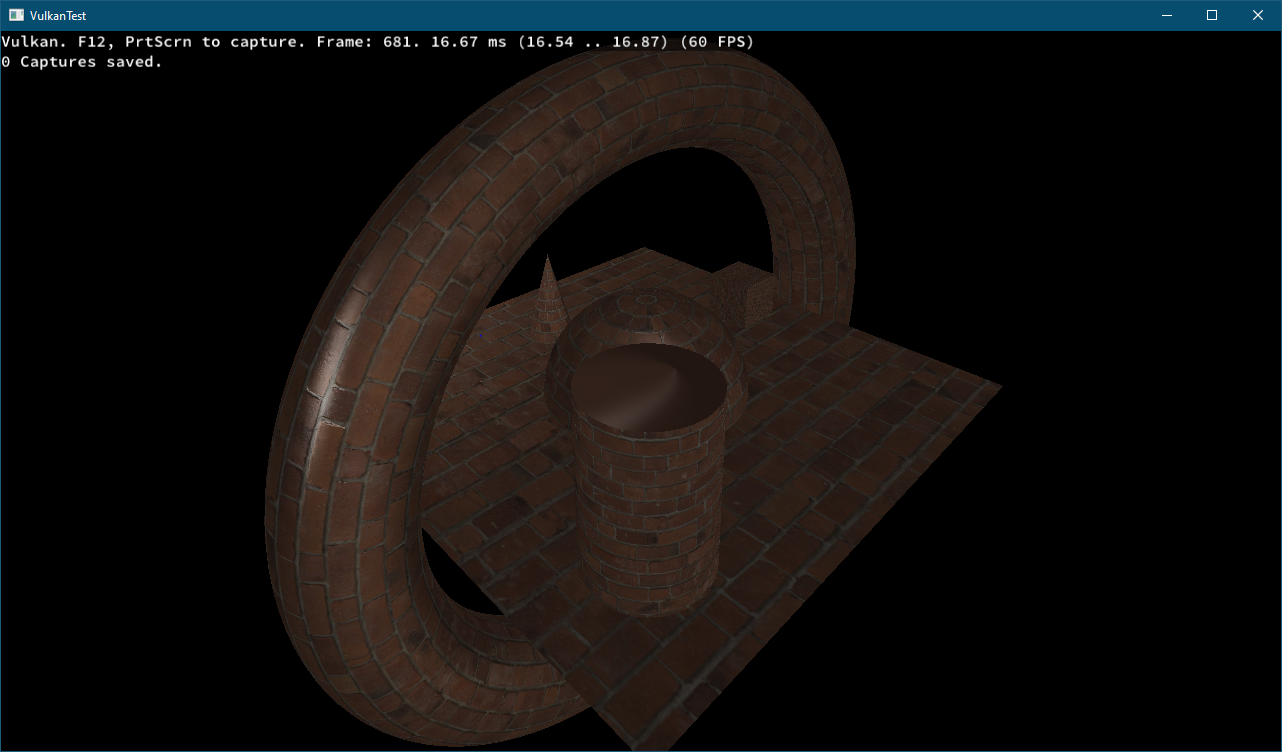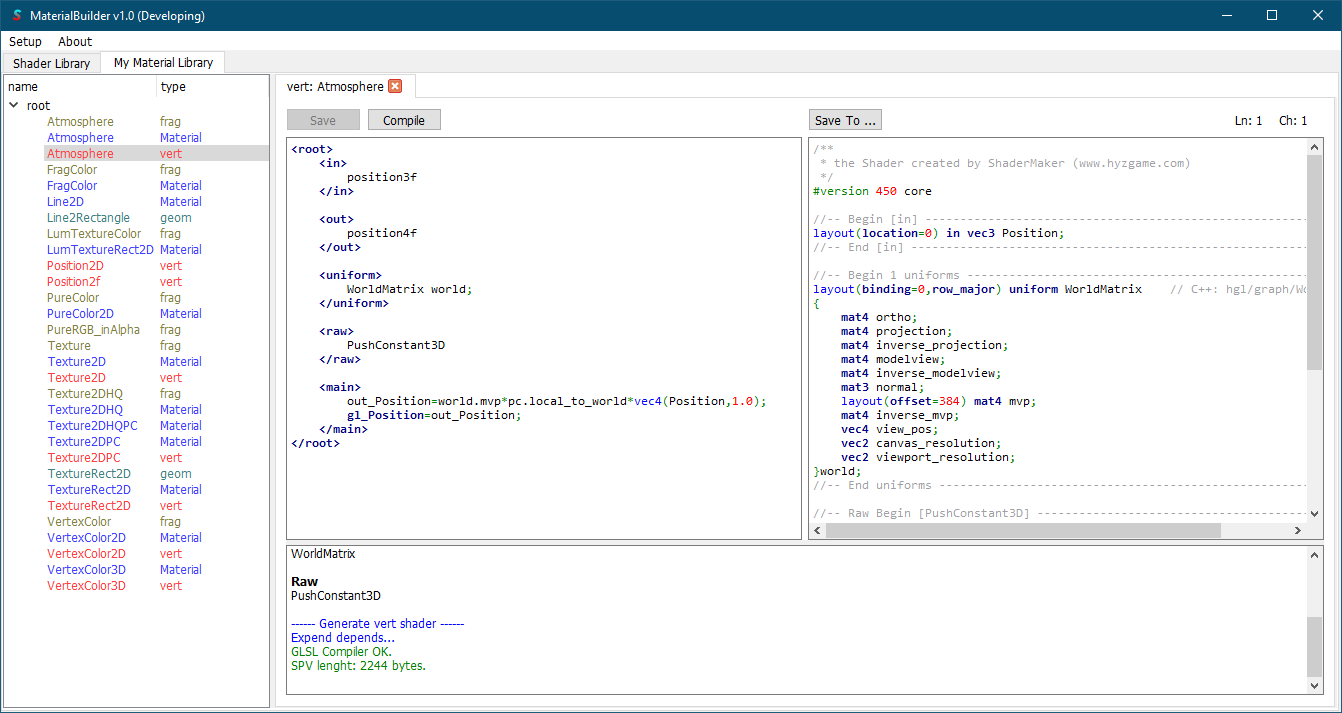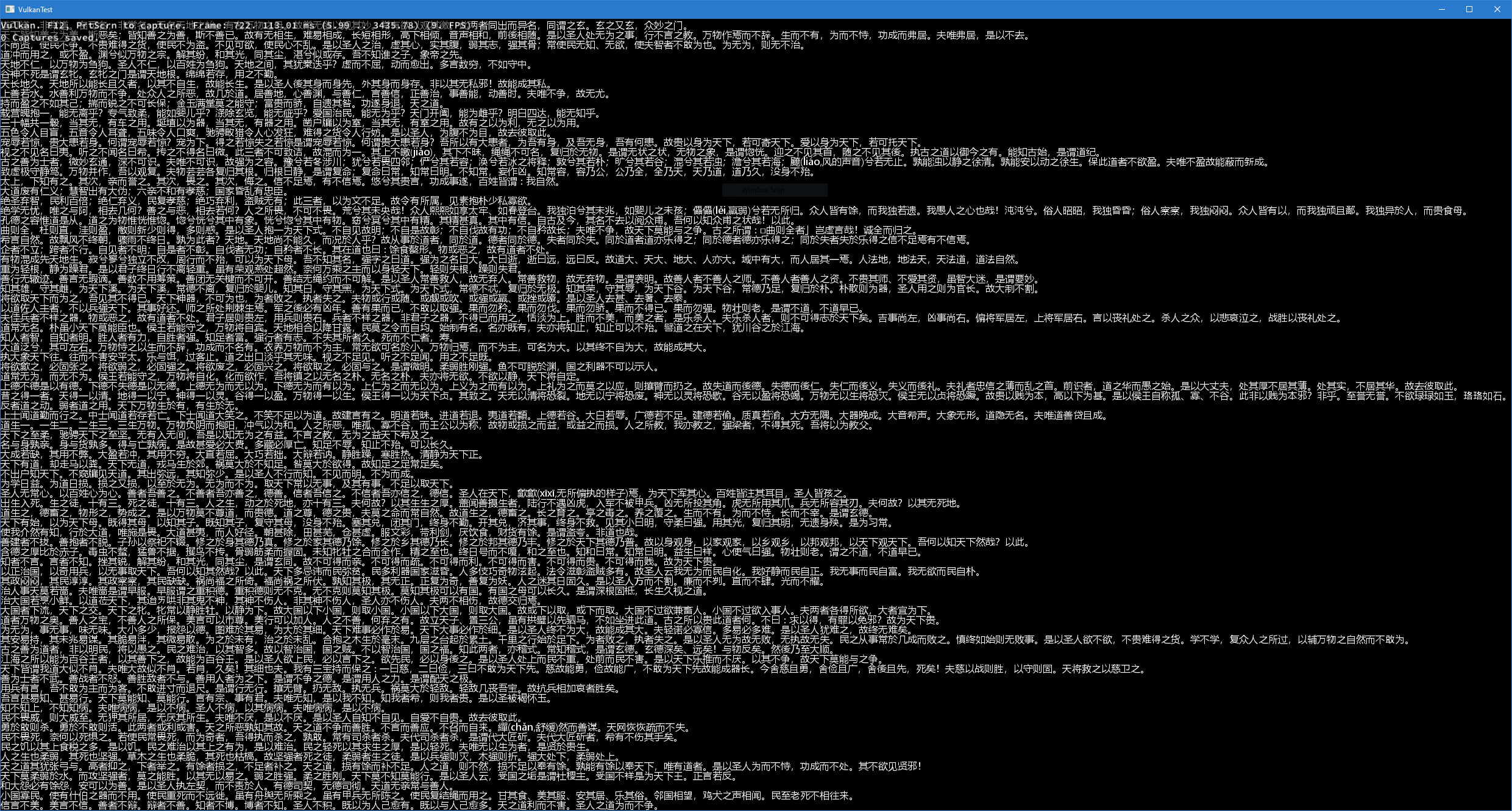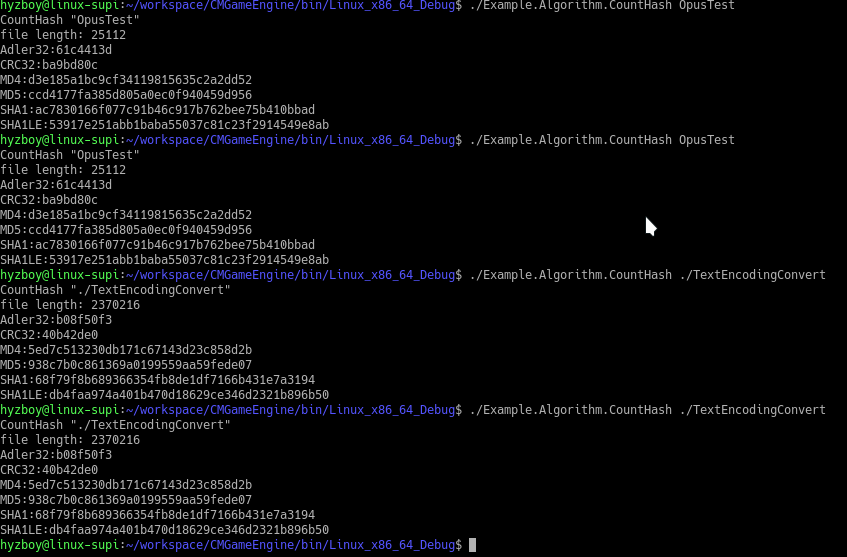﻿ 未分类 » 找寻那曾经失去的浪漫

# 存档在 ‘未分类’ 分类

## 2020年秋天的第一张延迟渲染

2020年10月22日## 新工具 MaterialEditor

2020年10月14日2020年10月8日

## 独立的GLSL编译工具

2020年9月5日

Github仓库

namespace glsl_compiler
{
enum class DescriptorType //等同VkDescriptorType
{
SAMPLER = 0,
COMBINED_IMAGE_SAMPLER = 1,
SAMPLED_IMAGE = 2,
STORAGE_IMAGE = 3,
UNIFORM_TEXEL_BUFFER = 4,
STORAGE_TEXEL_BUFFER = 5,
UNIFORM_BUFFER = 6,
STORAGE_BUFFER = 7,
UNIFORM_BUFFER_DYNAMIC = 8,
STORAGE_BUFFER_DYNAMIC = 9,
INPUT_ATTACHMENT = 10,

BEGIN_RANGE=SAMPLER,
END_RANGE=INPUT_ATTACHMENT,
RANGE_SIZE=(END_RANGE-BEGIN_RANGE)+1
};

enum class VertexAttribBaseType
{
Bool=0,
Int,
UInt,
Float,
Double,
};//enum class VertexAttribBaseType

{
char name;
uint8_t location;
uint32_t basetype; //对应enum class VertexAttribBaseType
uint32_t vec_size;
};//

{
uint32_t count;
};

{
char name;

uint8_t set;
uint8_t binding;
};

{
uint32_t count;
};

struct SPVData
{
bool result;
char *log;
char *debug_log;

uint32_t *spv_data;
uint32_t spv_length;

};

struct GLSLCompilerInterface
{
bool (*Init)(); ///<初始化
void (*Close)(); ///<关闭

SPVData * (*Compile)(const uint32_t type,const char *source); ///<编译glsl

void (*Free)(SPVData *); ///<释放数据
};

1. 加载动态链接库
2. 从动态链接库获取GetInterface函数
3. 用GetInterface函数得到一个GLSLCompilerInterface指针(以下简称GSI)
4. 调用GSI::Init函数
5. 自行加载glsl的文本，并保证为ansi或utf8字符集

• vert 顶点
• tesc 镶嵌控制
• tese 镶嵌评估
• geom 几何
• frag 片断
• comp 计算
• mesh 网格
8. 根据得到的SPVData::result来判断是否成功
• result为false表示失败，在log/debug_log中包含返回的错误信息
• result为true表示成功，在spv_data,spv_length中包含编译好的spv数据
9. 自行处理spv数据
10. 调用GSI::Free释放SPVData指针

## 文本显示，一个看起来很简单的功能

2020年8月4日## 现所有主要开源仓库一览

2020年4月5日
• 现今主力开发的超轻量级渲染引擎
• https://github.com/hyzboy/ULRE
• 曾经的旧主力引擎
• https://github.com/hyzboy/CMGameEngine
•  曾经短暂编写PHP网站时总结的小型PHP工具库
• https://github.com/hyzboy/CMPHP
• 修改的仅支持OpenGL Core的GLEW
• https://github.com/hyzboy/GLEWCore

## 2D/3D抛物线

2019年5月23日

#include<iostream>

using namespace std;
using uint=unsigned int;

struct vec2
{
float x,y;
};

struct vec3
{
float x,y,z;
};

/**
* 算出指定数量的抛物线高度值
* @param count 结果数量
* @param start 起始时间量，一般为-1
* @param end 结束时间量，一般为+1
*/
float *Parabolic(const uint count,const float time_start=-1,const float time_end=1)
{
if(count<=0)return(nullptr);

float *result=new float[count];

float rate;
float cur=time_start;
float total_time=time_end-time_start;

for(uint i=0;i<count;i++)
{
rate=float(i)/float(count);

cur=time_start+rate*total_time;

result[i]=1-cur*cur;

cout<<“height[“<<i<<“] “<<result[i]<<endl;
}

return result;
}

/**
* 画2D抛物线
* @param start 起始点
* @param end 结束点
* @param up 单位向上量
* @param height 高度值
* @param count 数量
*/
void Put2D(const vec2 &start,const vec2 &end,const vec2 &up,const float *height,const uint count)
{
vec2 total;
vec2 pos;
float rate;

total.x=end.x-start.x;
total.y=end.y-start.y;

cout<<“from “<<start.x<<“,”<<start.y<<” to “<<end.x<<“,”<<end.y<<endl;

for(uint i=0;i<count;i++)
{
rate=float(i)/float(count);

pos.x=start.x+rate*total.x;
pos.y=start.y+rate*total.y;

pos.x+=height[i]*up.x;
pos.y+=height[i]*up.y;

cout<<i<<“: “<<pos.x<<“,”<<pos.y<<endl;
}
}

/**
* 画3D抛物线
* @param start 起始点
* @param end 结束点
* @param up 单位向上量
* @param height 高度值
* @param count 数量
*/
void Put3D(const vec3 &start,const vec3 &end,const vec3 &up,const float *height,const uint count)
{
vec3 total;
vec3 pos;
float rate;

total.x=end.x-start.x;
total.y=end.y-start.y;
total.z=end.z-start.z;

cout<<“from “<<start.x<<“,”<<start.y<<“,”<<start.z<<” to “<<end.x<<“,”<<end.y<<“,”<<end.z<<endl;

for(uint i=0;i<count;i++)
{
rate=float(i)/float(count);

pos.x=start.x+rate*total.x;
pos.y=start.y+rate*total.y;
pos.z=start.z+rate*total.z;

pos.x+=height[i]*up.x;
pos.y+=height[i]*up.y;
pos.z+=height[i]*up.z;

cout<<i<<“: “<<pos.x<<“,”<<pos.y<<“,”<<pos.z<<endl;
}
}

void Test2D(float *height,const uint count)
{
vec2 start,end,up;

start.x=0;
start.y=0;

end.x=100;
end.y=0;

up.x=0;
up.y=100;

Put2D(start,end,up,height,count);
}

void Test3D(float *height,const uint count)
{
vec3 start,end,up;

start.x=0;
start.y=0;
start.z=0;

end.x=100;
end.y=0;
end.z=100;

up.x=0;
up.y=100;
up.z=0;

Put3D(start,end,up,height,count);
}

void main()
{
constexpr uint count=10;

float *height=Parabolic(count);

Test2D(height,count);
Test3D(height,count);

delete[] height;
}

## 重新支持C++Builder

2018年11月5日

Turbo C/C++、Borland C++、C++Builder曾经是我唯一的开发工具。在很久的一段时间里，我一直使用的是盗版，后来Borland公司的一位员工劝说我要使用正版。我同意了，我努力去做了，我也真的做到了。

## 新的strchr/strrchr/ClipFilename/ClipLastPathname函数

2018年8月22日

str: “hello,world!”
strchr(str,’,’) return “,world!”
strrchr(str,strlen(str),’,’) return “,world!”
strchr(str,”el”,2) return “ello,world!”
strrchr(str,strlen(str),”el”,2) return “ld!”
strrchr(str,strlen(str),3,’l’) return “lo,world!”
strrchr(str,strlen(str),3,”el”,2) return “lo,world!”
ClipFilename(“C:\1.txt”) return “1.txt”
ClipLastPathname(“C:\1\2”) return “2”
ClipLastPathname(“C:\1\2\”) return “2”
ClipLastPathname(“C:\1\2\\”) return “2”

## 新的HASH算法SHA1LE

2018年7月27日

SHA1LE算法是我们自定义的一种SHA1算法变种，它的计算方法与SHA1一致，改动如下:

1. 去掉了因BigEndian设计造成的LittleEndian处理器需要做大小头转换的部分
2. 可自定义起始因子
3. 可动态修改扰乱因子